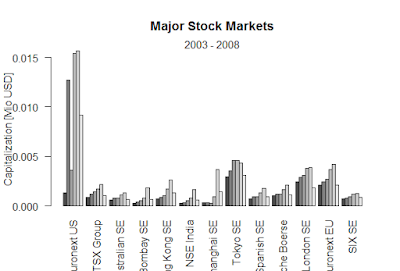Showing posts with label The co-movement with respect to the volatility between the three continuous return series. Show all posts
Showing posts with label The co-movement with respect to the volatility between the three continuous return series. Show all posts

## The co-movement with respect to the volatility between the three continuous return series

li b ra r y ( zoo ) 1
data ( EuStockMarkets ) 2
## Time Series plot of Levels
EuStockLevel <- as.zoo(EuStockMarkets)[,c("DAX", "CAC","FTSE")]
plot(EuStockLevel,xlab = "",main= "")

##Percentage returns
EuStockRet <- diff(log(EuStockLevel))*100
plot(EuStockRet,xlab = "",main= "")

## Cross correlation
layout(matrix(1:6,nrow = 3,ncol = 2,byrow = TRUE ))
ccf(EuStockRet[,1],EuStockRet[,2], ylab="",xlab="",lag.max = 20,main="Returns DAX vs CAC")
ccf(abs(EuStockRet)[, 1],abs(EuStockRet)[,2], ylab="",xlab="",lag.max = 20,main="Absolute returns DAX vs CAC")
ccf(EuStockRet[,1],EuStockRet[,3], ylab="", xlab="",lag.max = 20,main="Returns DAX vs FTSE")
ccf(abs(EuStockRet)[, 1],abs(EuStockRet)[,3], ylab="",xlab="",lag.max = 20,main="Absolute returns DAX vs FTSE")
ccf(EuStockRet[,2],EuStockRet[,3], ylab="",xlab="",lag.max = 20,main="Returns CAC vs FTSE")
ccf(abs(EuStockRet)[, 2],abs(EuStockRet)[,3], ylab="",xlab="",lag.max = 20,main="Absolute returns CAC vs FTSE")

## Rolling correlations
rollc <- function(x){dim <- ncol(x)
rcor <- cor(x)[lower.tri(diag(dim),diag = FALSE)]
return(rcor)
}
rcor<- rollapply(EuStockRet, width = 250, rollc,align = "right",by.column = FALSE)
colnames(rcor)<- c("DAX & CAC","DAX & FTSE","CAC & FTSE")

plot(rcor,main ="",xlab="")

### Black-Scholes formula-R

Black-Scholes formula-R > BlackScholes <- function(TypeFlag = c("c", "p"), S, X, Time, r, b, sigma) { TypeFla...